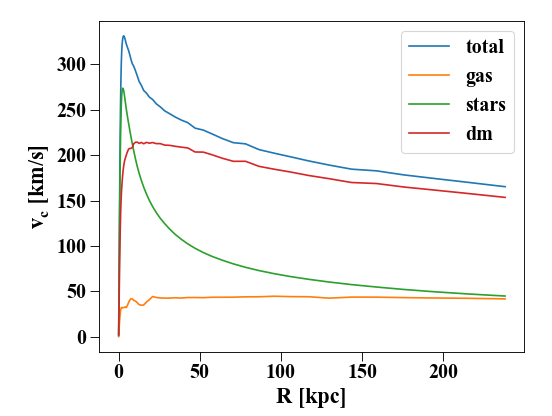# Plotting Rotation Cuves¶

Rotation curves are calculated and plotted using the `Profile()` object. For a tutorial on profiles see Profiles in Pynbody.

```import pynbody
import matplotlib.pylab as plt
from os import environ
# load the snapshot and set to physical units

h = s.halos()

# center on the largest halo and align the disk
pynbody.analysis.angmom.faceon(h)

# create a profile object for the stars
s.physical_units()
p = pynbody.analysis.profile.Profile(h,rmin=.01,rmax =250,type='log',ndim=3)
pg = pynbody.analysis.profile.Profile(h.g,rmin=.01,rmax =250,type='log',ndim=3)
ps = pynbody.analysis.profile.Profile(h.s,rmin=.01,rmax =250,type='log',ndim=3)
pd = pynbody.analysis.profile.Profile(h.d,rmin=.01,rmax =250,type='log',ndim=3)

# make the plot
plt.plot(p['rbins'],p['v_circ'],label='total')
plt.plot(pg['rbins'],pg['v_circ'],label='gas')
plt.plot(ps['rbins'],ps['v_circ'],label='stars')
plt.plot(pd['rbins'],pd['v_circ'],label='dm')

plt.xlabel('R [kpc]')
plt.ylabel(r'\$v_c\$ [km/s]')
plt.legend()
```## Speeding up the Gravity Calculation in Pynbody¶

The rotation curve is calculated by calculating the forces in a plane. The force calculation is a directcalculation, so it takes a while and it is therefore done in C. It is even faster if you use the parallel Open-MP version. This was installed automatically if pynbody detected an Open-MP C compiler during setup. To see whether this happened or not, you can ask how many cores your machine has:

```In : import pynbody

In : pynbody.openmp.get_cpus()
Out: 1
```

If this returns 1 but you have more than one core, pynbody has not been installed with OpenMP support (check your compiler, especially on Mac OS - then raise an issue if you can’t fix the problem) . If it returns the number of cores on your machine, you’re in luck.

Assuming OpenMP support is enabled, the actual number of cores used by pynbody is determined by the configuration option `number_of_threads`, which is the number of CPUs detected on your machine by default. If you want to reduce this (e.g. you are running on a login node or have multiple analyses going on in parallel), you can specify the number of cores explicitly:

```In : pynbody.config['number_of_threads'] = 2
```

Now all gravity calculations will use the parallel gravity calculation on only 2 cpus. Note that the number of threads you specify in this option will also be the default for other routines, such as `pynbody.plot.sph.image()`. See Use of multiple processors by pynbody.

If you want to make your configuration changes permanent, create a file called `.pynbodyrc` in your home directory with the lines

```[general]